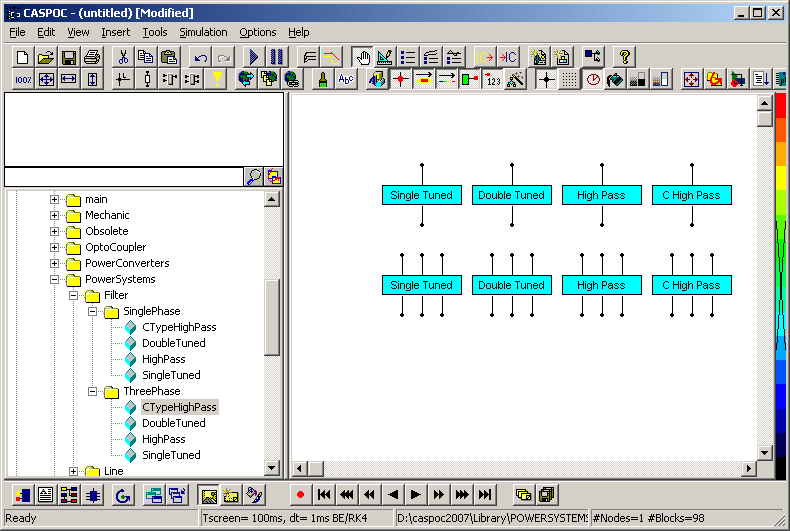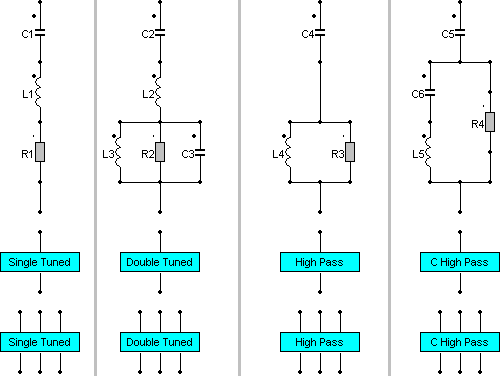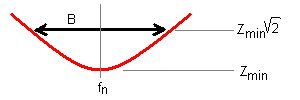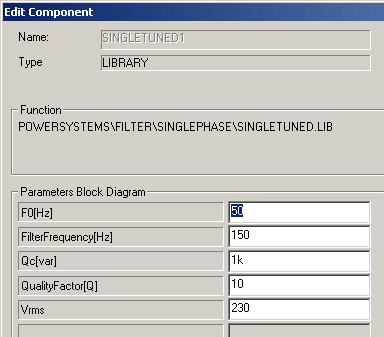• Introduction
• What is in this manual
• What is Caspoc
• User interface
• Introduction
• Starting
• Simulation
• Editing
• Viewing and printing
• Getting Started
• Basic editing
• Simulation in the time domain
• Basic User Interface Topics
• Editing
• Simulation
• Viewing
• Library
• Reports
• Project management
• Circuit and Block Diagram Components
• Introduction
• Cscript and user defined functions
• Component parameters
• Modeling Topics
• Introduction
• Power Electronics
• Semiconductors
• Electrical Machines
• Electrical drives
• Power Systems
• Mechanical Systems
• Thermal Systems
• Magnetic Circuits
• Green Energy
• Coupling to FEM
• Experimenter
• Analog hardware description language
• Embedded C code Export
• Coupling to Spice
• Small Signal Analysis
• Matlab coupling
• Tips and tricks
• Appendices

## Harmonic Filters Single and Three Phase.

In Caspoc filters are constructed from R, L and C circuit components. In power systems it is convenient to have ready models for filters, both single and three phase where you can directly specify the filter frequency, quality and reactive power at nominal voltage.Three-phase harmonic filters are shunt elements that are used in power systems for decreasing voltage distortion and for power factor correction. Nonlinear elements such as power electronic converters generate harmonic currents or harmonic voltages, which are injected into power system. The resulting distorted currents flowing through system impedance produce harmonic voltage distortion. Harmonic filters reduce distortion by diverting harmonic currents in low impedance paths. Harmonic filters are designed to be capacitive at fundamental frequency, so that they are also used for producing reactive power required by converters and for power factor correction.

In order to achieve an acceptable distortion, several banks of filters of different types are usually connected in parallel. The most commonly used filter types are:

• Band-pass filters, which are used to filter lowest order harmonics such as 5th, 7th, 11th, 13th, etc. Band-pass filters can be tuned at a single frequency (single-tuned filter) or at two frequencies (double-tuned filter).
• High-pass filters, which are used to filter high-order harmonics and cover a wide range of frequencies. A special type of high-pass filter, the C-type high-pass filter, is used to provide reactive power and avoid parallel resonances. It also allows filtering low order harmonics (such as 3rd), while keeping zero losses at fundamental frequency.

The Three-Phase Harmonic Filter is built of RLC elements. The resistance, inductance, and capacitance values are determined from the filter type and from the following parameters:

• Reactive power at nominal voltage
• Tuning frequencies
• Quality factor. The quality factor is a measure of the sharpness of the tuning frequency. It is determined by the resistance value.

The four types of filters that can be modeled with the Three-PhaseHarmonic Filter block are shown below:The simplest filter type is the single-tuned filter. The following figure gives the definition of the quality factor Q and practical formulae for computing the reactive power QC and losses (active power P). The quality factor Q of the filter is the quality factor of the reactance at the tuning frequency Q = (n.XL)/R. The quality factor determines the bandwidth B=fn/Q, which is a measure of the sharpness of the tuning frequency as shown below.where:
• f1=Fundamental frequency
• ω=2πf1 = Angular frequency
• fn=Tuning frequency
• n=Harmonic orderfn/f1
• V=Nominal line-line voltage
• XL=Inductor reactance at fundamental frequency
• XC=Capacitor reactance at fundamental frequency

The double-tuned filter performs the same function as two single-tuned filters although it has certain advantages: its losses are much lower and the impedance magnitude at the frequency of the parallel resonance that arises between the two tuning frequencies is lower.

The double-tuned filter consists of a series LC circuit and a parallel RLC circuit. If f1 and f2 are the two tuning frequencies, both the series circuit and the parallel circuit are tuned to approximately the mean geometric frequency

fm=SQRT(f1f2)

The quality factor Q of the double-tuned filter is defined as the quality factor of the parallel L, R elements at the mean frequency fm:

Q=R/ (L2πfm)

The high-pass filter is a single-tuned filter where the L and R elements are connected in parallel instead of series. This connection results in a wide-band filter having an impedance at high frequencies limited by the resistance R.

The quality factor of the high-pass filter is the quality factor of the parallel RL circuit at the tuning frequency:

Q=R/ (L2πfn)

The C-type high-pass filter is a variation of the high-pass filter, where the inductance L is replaced with a series LC circuit tuned at the fundamental frequency. At fundamental frequency, the resistance is, therefore, bypassed by the resonant LC circuit and losses are null.

The quality factor of the C-type filter is still given by the ratio:

Q=R/ (L2πfm)

## ParametersNominal L-L voltage and frequency The nominal phase-to-phase voltage of the filter, in volts RMS (Vrms) and the nominal frequency, in hertz (F0). Nominal reactive power The three-phase capacitive reactive power QC, in vars. Specify a positive value. Tuning frequency or Tuning frequencies The tuning filter frequency of the single frequency filter (single-tuned, high-pass or C-type high-pass), or the two frequencies of the double-tuned filter, in hertz (Hz). Quality factor (Q) The quality factor Q of the filter defined as explained above for each typical filter. Dimensionless positive number.